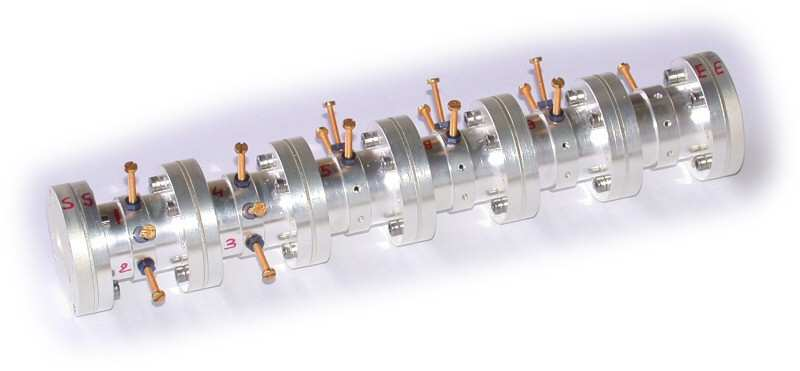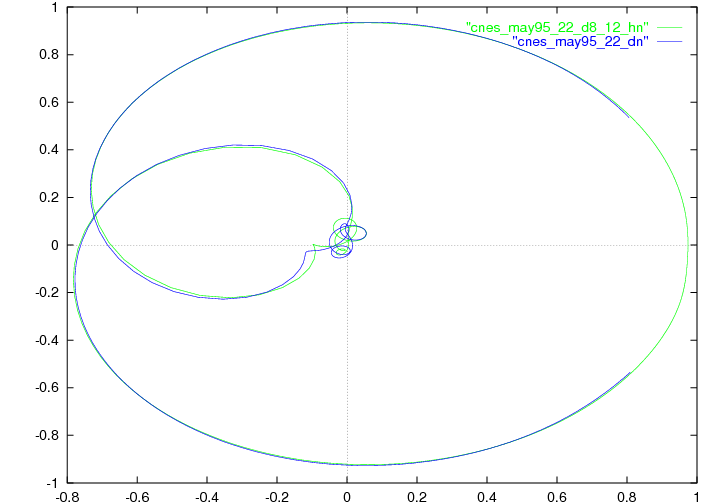EN FR
EN FR
##### APICS - 2016
Overall Objectives
Bilateral Contracts and Grants with Industry
Bibliography
Overall Objectives
Bilateral Contracts and Grants with Industry
Bibliography

## Section: Application Domains

### Identification and design of microwave devices

Participants : Laurent Baratchart, Sylvain Chevillard, Jean-Paul Marmorat, Martine Olivi, Fabien Seyfert.

This is joint work with Stéphane Bila (XLIM, Limoges).

One of the best training grounds for function-theoretic applications by the team is the identification and design of physical systems whose performance is assessed frequency-wise. This is the case of electromagnetic resonant systems which are of common use in telecommunications.

In space telecommunications (satellite transmissions), constraints specific to on-board technology lead to the use of filters with resonant cavities in the microwave range. These filters serve multiplexing purposes (before or after amplification), and consist of a sequence of cylindrical hollow bodies, magnetically coupled by irises (orthogonal double slits). The electromagnetic wave that traverses the cavities satisfies the Maxwell equations, forcing the tangent electrical field along the body of the cavity to be zero. A deeper study of the Helmholtz equation states that an essentially discrete set of wave vectors is selected. In the considered range of frequency, the electrical field in each cavity can be decomposed along two orthogonal modes, perpendicular to the axis of the cavity (other modes are far off in the frequency domain, and their influence can be neglected).

Figure 1. Picture of a 6-cavities dual mode filter. Each cavity (except the last one) has 3 screws to couple the modes within the cavity, so that 16 quantities must be optimized. Quantities such as the diameter and length of the cavities, or the width of the 11 slits are fixed during the design phase.Each cavity (see Figure 1) has three screws, horizontal, vertical and midway (horizontal and vertical are two arbitrary directions, the third direction makes an angle of 45 or 135 degrees, the easy case is when all cavities show the same orientation, and when the directions of the irises are the same, as well as the input and output slits). Since screws are conductors, they behave as capacitors; besides, the electrical field on the surface has to be zero, which modifies the boundary conditions of one of the two modes (for the other mode, the electrical field is zero hence it is not influenced by the screw), the third screw acts as a coupling between the two modes. The effect of an iris is opposite to that of a screw: no condition is imposed on a hole, which results in a coupling between two horizontal (or two vertical) modes of adjacent cavities (in fact the iris is the union of two rectangles, the important parameter being their width). The design of a filter consists in finding the size of each cavity, and the width of each iris. Subsequently, the filter can be constructed and tuned by adjusting the screws. Finally, the screws are glued once a satisfactory response has been obtained. In what follows, we shall consider a typical example, a filter designed by the CNES in Toulouse, with four cavities near 11 GHz.

Near the resonance frequency, a good approximation to the Helmholtz equations is given by a second order differential equation. Thus, one obtains an electrical model of the filter as a sequence of electrically-coupled resonant circuits, each circuit being modeled by two resonators, one per mode, the resonance frequency of which represents the frequency of a mode, and whose resistance accounts for electric losses (surface currents) in the cavities.

This way, the filter can be seen as a quadripole, with two ports, when plugged onto a resistor at one end and fed with some potential at the other end. One is now interested in the power which is transmitted and reflected. This leads one to define a scattering matrix $S$, which may be considered as the transfer function of a stable causal linear dynamical system, with two inputs and two outputs. Its diagonal terms ${S}_{1,1}$, ${S}_{2,2}$ correspond to reflections at each port, while ${S}_{1,2}$, ${S}_{2,1}$ correspond to transmission. These functions can be measured at certain frequencies (on the imaginary axis). The matrix $S$ is approximately rational of order 4 times the number of cavities (that is 16 in the example on Figure 2), and the key step consists in expressing the components of the equivalent electrical circuit as functions of the ${S}_{ij}$ (since there are no formulas expressing the lengths of the screws in terms of parameters of this electrical model). This representation is also useful to analyze the numerical simulations of the Maxwell equations, and to check the quality of a design, in particular the absence of higher resonant modes.

In fact, resonance is not studied via the electrical model, but via a low-pass equivalent circuit obtained upon linearizing near the central frequency, which is no longer conjugate symmetric (i.e. the underlying system may no longer have real coefficients) but whose degree is divided by 2 (8 in the example).

In short, the strategy for identification is as follows:

• measuring the scattering matrix of the filter near the optimal frequency over twice the pass band (which is 80MHz in the example).

• Solving bounded extremal problems for the transmission and the reflection (the modulus of he response being respectively close to 0 and 1 outside the interval measurement, cf. Section 3.3.1) in order to get a models for the scattering matrix as an analytic matrix-valued function. This provides us with a scattering matrix known to be close to a rational matrix of order roughly 1/4 of the number of data points.

• Approximating this scattering matrix by a true rational transfer-function of appropriate degree (8 in this example) via the Endymion or RARL2 software (cf. Section 3.3.2.2).

• A state space realization of $S$, viewed as a transfer function, can then be obtained, where additional symmetry constraints coming from the reciprocity law and possibly other physical features of the device have to be imposed.

• Finally one builds a realization of the approximant and looks for a change of variables that eliminates non-physical couplings. This is obtained by using algebraic-solvers and continuation algorithms on the group of orthogonal complex matrices (symmetry forces this type of transformation).

Figure 2. Nyquist Diagram. Rational approximation (degree 8) and data - ${S}_{22}$.The final approximation is of high quality. This can be interpreted as a confirmation of the linearity assumption on the system: the relative ${L}^{2}$ error is less than ${10}^{-3}$. This is illustrated by a reflection diagram (Figure 2). Non-physical couplings are less than ${10}^{-2}$.

The above considerations are valid for a large class of filters. These developments have also been used for the design of non-symmetric filters, which are useful for the synthesis of repeating devices.

The team further investigates problems relative to the design of optimal responses for microwave devices. The resolution of a quasi-convex Zolotarev problems was proposed, in order to derive guaranteed optimal multi-band filter responses subject to modulus constraints . This generalizes the classical single band design techniques based on Chebyshev polynomials and elliptic functions. The approach relies on the fact that the modulus of the scattering parameter $|{S}_{1,2}|$ admits a simple expression in terms of the filtering function $D=|{S}_{1,1}|/|{S}_{1,2}|$, namely

$|{S}_{1,2}{|}^{2}=\frac{1}{1+{D}^{2}}.$

The filtering function appears to be the ratio of two polynomials ${p}_{1}/{p}_{2}$, the numerator of the reflection and transmission scattering factors, that may be chosen freely. The denominator $q$ is then obtained as the unique stable unitary polynomial solving the classical Feldtkeller spectral equation:

$q{q}^{*}={p}_{1}{p}_{1}^{*}+{p}_{2}{p}_{2}^{*}.$

The relative simplicity of the derivation of a filter's response, under modulus constraints, owes much to the possibility of forgetting about Feldtkeller's equation and express all design constraints in terms of the filtering function. This no longer the case when considering the synthesis $N$-port devices for $N>3$, like multiplexers, routers and power dividers, or when considering the synthesis of filters under matching conditions. The efficient derivation of multiplexers responses is the subject of recent investigation by Apics, using techniques based on constrained Nevanlinna-Pick interpolation (see Section 5.2).

Through contacts with CNES (Toulouse) and UPV (Bilbao), Apics got additionally involved in the design of amplifiers which, unlike filters, are active devices. A prominent issue here is stability. A twenty years back, it was not possible to simulate unstable responses, and only after building a device could one detect instability. The advent of so-called harmonic balance techniques, which compute steady state responses of linear elements in the frequency domain and look for a periodic state in the time domain of a network connecting these linear elements via static non-linearities made it possible to compute the harmonic response of a (possibly nonlinear and unstable) device . This has had tremendous impact on design, and there is a growing demand for software analyzers. The team is also becoming active in this area.

In this connection, there are two types of stability involved. The first is stability of a fixed point around which the linearized transfer function accounts for small signal amplification. The second is stability of a limit cycle which is reached when the input signal is no longer small and truly nonlinear amplification is attained (e.g. because of saturation). Work by the team so far has been concerned with the first type of stability, and emphasis is put on defining and extracting the “unstable part” of the response, see Section 5.4. The stability check for limit cycles is now under investigation.